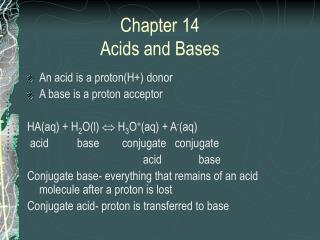DownloadDownload PresentationChapter 14 Acids and Bases

# Chapter 14 Acids and Bases

Télécharger la présentation## Chapter 14 Acids and Bases

- - - - - - - - - - - - - - - - - - - - - - - - - - - E N D - - - - - - - - - - - - - - - - - - - - - - - - - - -
##### Presentation Transcript

1. Chapter 14Acids and Bases • An acid is a proton(H+) donor • A base is a proton acceptor HA(aq) + H2O(l)  H3O+(aq) + A-(aq) acid base conjugate conjugate acid base Conjugate base- everything that remains of an acid molecule after a proton is lost Conjugate acid- proton is transferred to base

2. Acid dissociation constant What is the equilibrium expression for the general reaction? Ka= [H3O+] [A-]/[HA] or [H+] [A-]/[HA] Ka is called the acid dissociation constant

3. Acid Strength Strong acid- equilibrium lies far to the right Ka is large HCl, H2SO4, HNO4, HClO4 Weak acid- equilibrium lies far to the left Ka is small Phosphoric acid, Nitrous acid, Acetic acid

4. Water as an acid and a base • Amphoteric- acts as either an acid or a base • Autoionization of water-transfer of a proton from 1 H20 molecule to another to produce hydroxide ion and the hydronium ion H2O + H2O  H3O+ + OH- or 2H20(l)  H3O+ (aq) + OH-(aq) This leads to the Ion-product constant,Kw

5. Kw con. Kw= [H3O+] [OH-] or [H+] [OH-] At 25° C, [H+] = [OH-]= 1.0x10-7M Kw= (1.0x10-7) (1.0x10-7)= 1.0x10-14 At 25° C no matter what it contains, the product of [H+] and [OH-] 1.0x10-14

6. 3 Possible situations • Neutral solution [H+] = [OH-] • Acid solution [H+]  [OH-] • Basic solution [H+] [OH-] Calculate [H+] or [OH-] at 25°C. State if neutral, acidic, or basic. • 1.0x10-5M OH- • 1.0x10-7M OH- • 10.0 M H+

7. pH Scale pH= -log[H+] Example: [H+]=1.0x10-7M pH= 7.00 Other Log Scales pOH= -log[OH-] pK= -logK

8. Example14.5 Calculate pH and pOH for the following at 25°C. • 1.0x10-3 M OH • 1.0 M H+

9. Converting between p and concentration • pX= -log[X]  (pH or pOH) = -log[H+ or OH-] • [X] = 10-pX  [H+ or OH-] = 10-pX

10. Calculate “p” or [ ] of the following: • Calculate [Cl-] if pCl = 7.32 • Calculate pAg if [Ag+] = .034 M The pH of a sample of blood equals 7.41 at 25°C. Calculate the pOH, [H+], and [OH-]

11. Calculating pH of Strong Acid Solutions What are some examples of strong acids? HCl, HNO3, H2SO4, and HClO4 Calculate the pH and [OH-] of a 5 x10-3 M HClO4 solution. Calculate the pH of 1.0 x10-10 M HCl.

12. Calculating the pH of a Weak Acid Calculate the pH of a .100M aqueous solution of hypochlorous acid(HOCl) where Ka= 3.5x10-8. • List the major species. HOCl H2O

13. Con. • Write the balanced equations for the reactions producing H+. HOCl(aq) H+(aq) + OCl-(aq) H2O(l)  H+(aq) + OH-(aq) • Compare equilibrium constants to determine the dominate H+ Ka= 3.5x10-8 Kw= 1.0x10-14

14. Con. • Write the equilibrium expression Ka= [H+] [OCl-]/ [HOCl] • Do an ICEbox

15. Con. • Substitute in Ka 3.5x10-8 = [x] [x] / [.100-x] 3.5x10-8 = x2/ .100 x= 5.9 x 10-5

16. Con. • Validate the approximation by 5% - compare x to [HA]0 x100% 5.95 x10-5/.100 x100= .059% • Calculate [H+] and pH [H+]= 5.95x10-5 M pH= -log[5.95x10-5] = 4.225

17. pH of a Weak Acid Mixtures • Calculate the pH of a solution that contains 1.00 M HCN(Ka=6.2x10-10) and 5.00 M HNO2 (Ka= 4.0x10-4). HCN(aq)H+(aq) + CN-(aq) Ka= 6.2x10-10 HNO2(aq)  H+(aq) + NO2-(aq) Ka= 4.0 x10-4 H2O(l)  H+(aq) + OH-(aq) Kw= 1.0x10-14

18. Con. Ka= [H+] [NO2-]/ [HNO2] HNO2 H+ NO2- I 5.00 0 0 C -x +x +x E 5.00 –x x x

19. Con. 4.0 x10-4 = [x] [x]/ 5.00-x approximate so 4.0 x10-4 = x2/5.00 X=4.5 x10-2 Compare: 4.5 x10-2 /5.00 x100 = .90% [H+]= 4.5 x10-2 M pH= -log[4.5 x10-2 ] = 1.35

20. Bases • Proton acceptors • Examples of strong bases: • NaOH, KOH, all groups in 1A&IIA